# ML Aggarwal Solutions for Class 9 Maths Chapter 8 Indices

ML Aggarwal Solutions for Class 9 Maths Chapter 8 Indices is a useful resource for students to understand and prepare for the ICSE board examinations. These solutions help students obtain a clear conceptual knowledge of all the chapters of ML Aggarwal textbooks and also help them solve problems in an effective manner. Further, the experts at BYJU’S have provided the ML Aggarwal Solutions for Class 9 Maths Chapter 8 Indices PDF, in order to help students with their exam preparation.

The 8th chapter contains problems on simplifying the powers and exponents of numbers and algebraic expressions. The solutions can be utilised by students for any doubt clearance or quick reference during the process of self-study. The ML Aggarwal solutions are primarily created to help students with their exam preparations and boost their confidence in solving any difficult problems. All these solutions are according to the latest ICSE guidelines thus, ensuring the high possibilities of securing excellent marks in the examinations.

## ML Aggarwal Solutions for Class 9 Maths Chapter 8 Indices Download PDF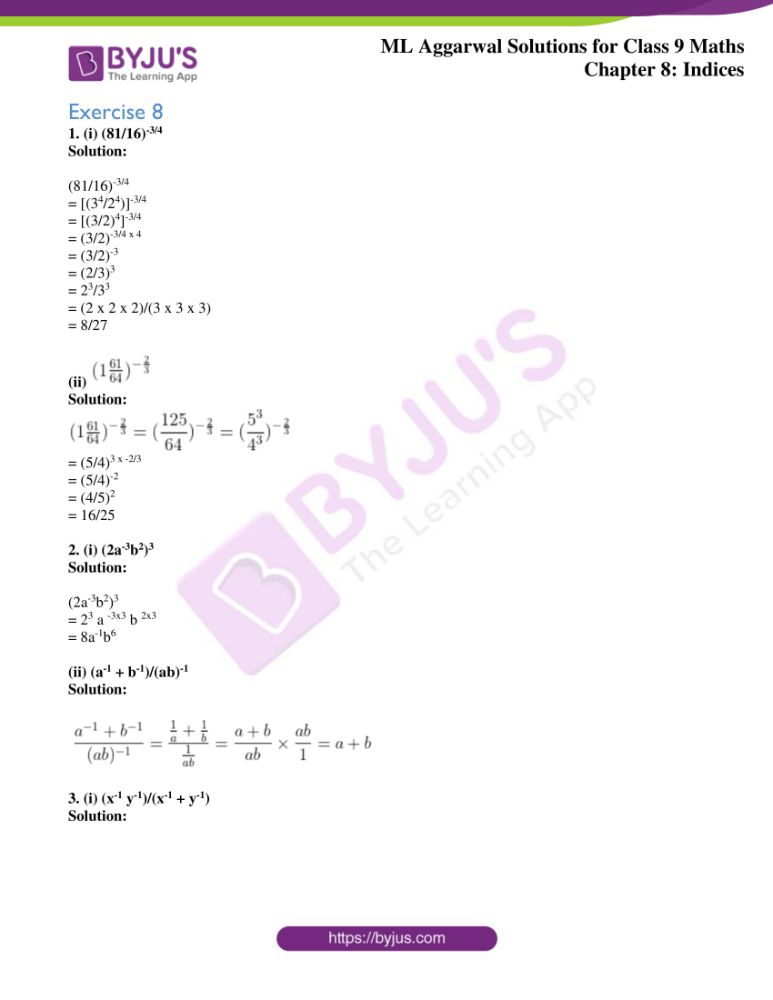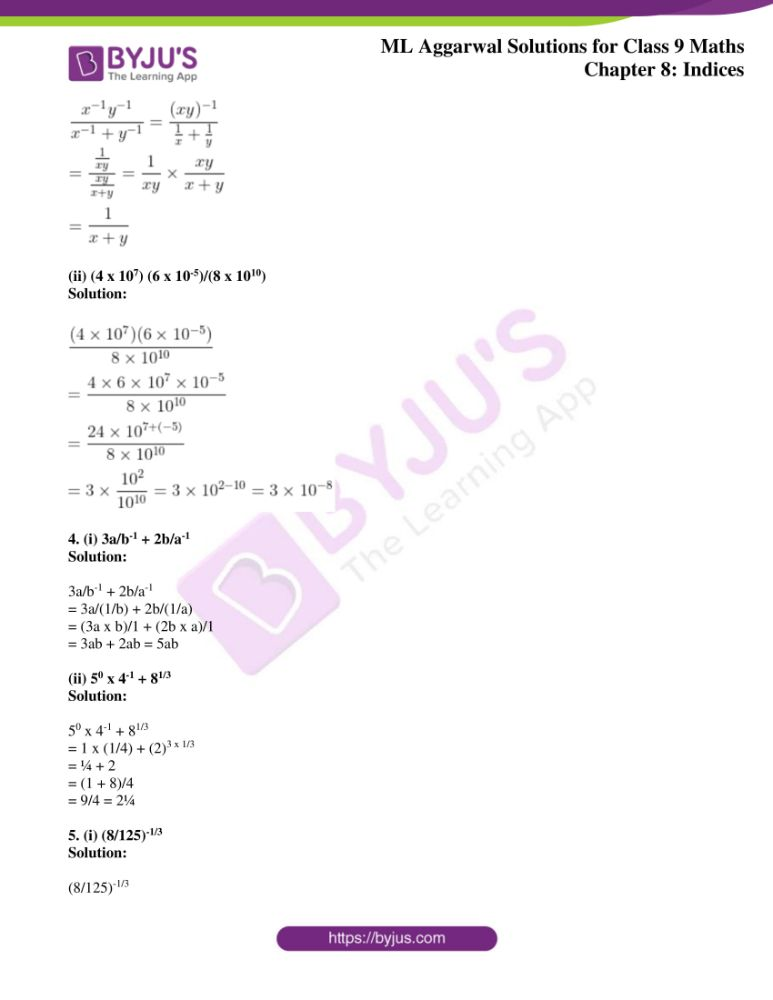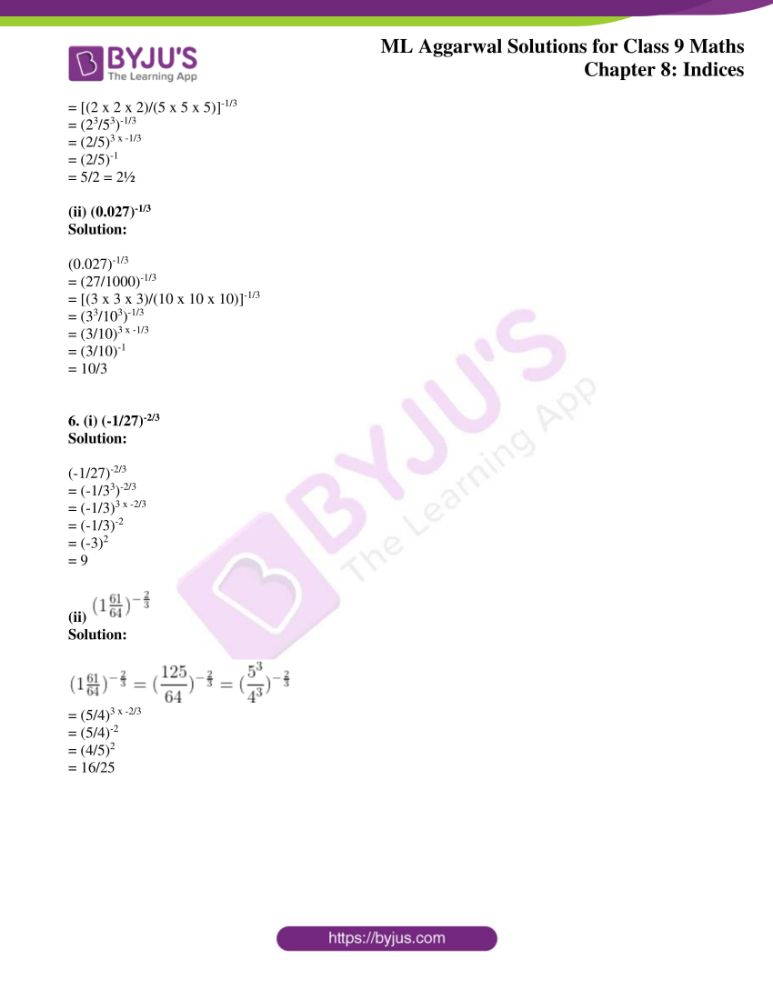## Access ML Aggarwal Solutions for Class 9 Maths Chapter 8 Indices

1. (i) (81/16)-3/4

Solution:

(81/16)-3/4

= [(34/24)]-3/4

= [(3/2)4]-3/4

= (3/2)-3/4 x 4

= (3/2)-3

= (2/3)3

= 23/33

= (2 x 2 x 2)/(3 x 3 x 3)

= 8/27

(ii)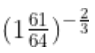Solution: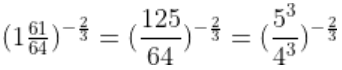= (5/4)3 x -2/3

= (5/4)-2

= (4/5)2

= 16/25

2. (i) (2a-3b2)3

Solution:

(2a-3b2)3

= 23 a -3×3 b 2×3

= 8a-1b6

(ii) (a-1 + b-1)/(ab)-1

Solution: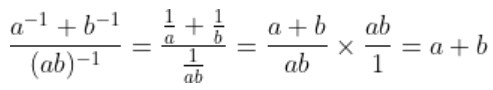3. (i) (x-1 y-1)/(x-1 + y-1)

Solution: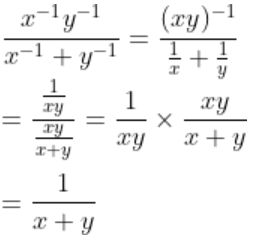(ii) (4 x 107) (6 x 10-5)/(8 x 1010)

Solution: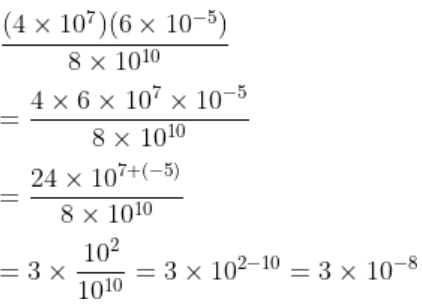4. (i) 3a/b-1 + 2b/a-1

Solution:

3a/b-1 + 2b/a-1

= 3a/(1/b) + 2b/(1/a)

= (3a x b)/1 + (2b x a)/1

= 3ab + 2ab = 5ab

(ii) 50 x 4-1 + 81/3

Solution:

50 x 4-1 + 81/3

= 1 x (1/4) + (2)3 x 1/3

= ¼ + 2

= (1 + 8)/4

= 9/4 = 2¼

5. (i) (8/125)-1/3

Solution:

(8/125)-1/3

= [(2 x 2 x 2)/(5 x 5 x 5)]-1/3

= (23/53)-1/3

= (2/5)3 x -1/3

= (2/5)-1

= 5/2 = 2½

(ii) (0.027)-1/3

Solution:

(0.027)-1/3

= (27/1000)-1/3

= [(3 x 3 x 3)/(10 x 10 x 10)]-1/3

= (33/103)-1/3

= (3/10)3 x -1/3

= (3/10)-1

= 10/3

6. (i) (-1/27)-2/3

Solution:

(-1/27)-2/3

= (-1/33)-2/3

= (-1/3)3 x -2/3

= (-1/3)-2

= (-3)2

= 9

(ii)Solution:= (5/4)3 x -2/3

= (5/4)-2

= (4/5)2

= 16/25